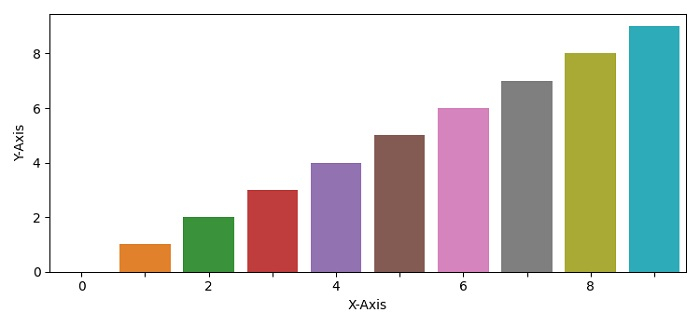# How to decrease the density of x-ticks in Seaborn?

MatplotlibPythonData Visualization

#### Python Data Science basics with Numpy, Pandas and Matplotlib

Most Popular

63 Lectures 6 hours

#### Data Visualization using MatPlotLib & Seaborn

11 Lectures 4 hours

#### MatPlotLib with Python

9 Lectures 2.5 hours

To decrease the density of x-ticks in Seaborn, we can use set_visible=False for odd positions.

## Steps

• Set the figure size and adjust the padding between and around the subplots.

• Create a dataframe with X-axis and Y-axis keys.

• Show the point estimates and confidence intervals with bars, using barplot() method.

• Iterate bar_plot.get_xticklabels() method. If index is even, then make them visible; else, not visible.

• To display the figure, use show() method.

## Example

import pandas
import matplotlib.pylab as plt
import seaborn as sns
plt.rcParams["figure.figsize"] = [7.50, 3.50]
plt.rcParams["figure.autolayout"] = True
df = pandas.DataFrame({"X-Axis": [i for i in range(10)], "Y-Axis": [i for i in range(10)]})
bar_plot = sns.barplot(x='X-Axis', y='Y-Axis', data=df)
for index, label in enumerate(bar_plot.get_xticklabels()):
if index % 2 == 0:
label.set_visible(True)
else:
label.set_visible(False)
plt.show()

## Output